# Few Selected Problems Chapter 4 1 From base

• Slides: 74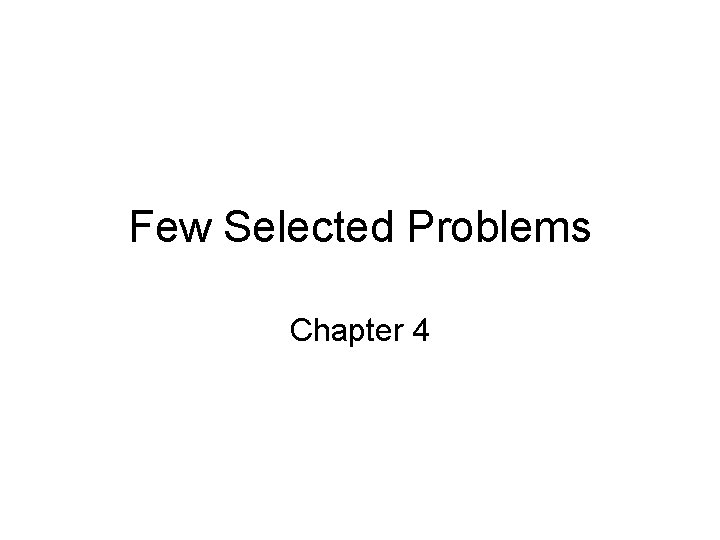Few Selected Problems Chapter 4• 1. From base price levels of 100 in 2000, Japanese and U. S. price levels in 2003 stood at 102 and 106, respectively. – a. If the 2000 \$: ¥ exchange rate was \$0. 007692, what should the exchange rate be in 2003?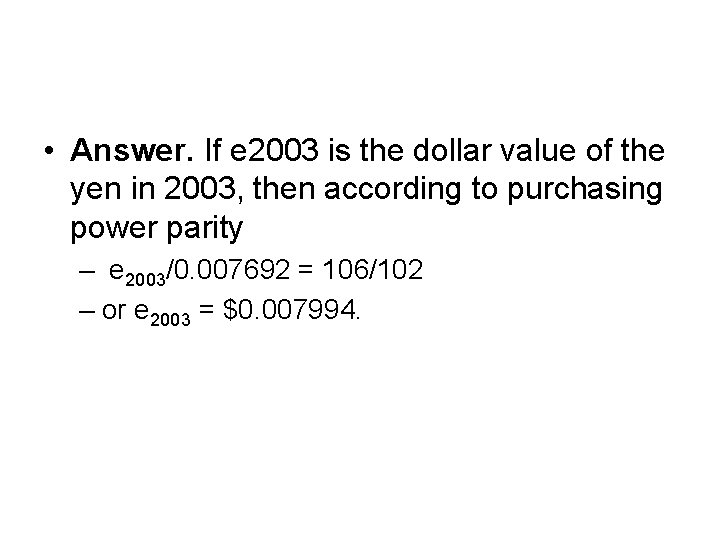• Answer. If e 2003 is the dollar value of the yen in 2003, then according to purchasing power parity – e 2003/0. 007692 = 106/102 – or e 2003 = \$0. 007994.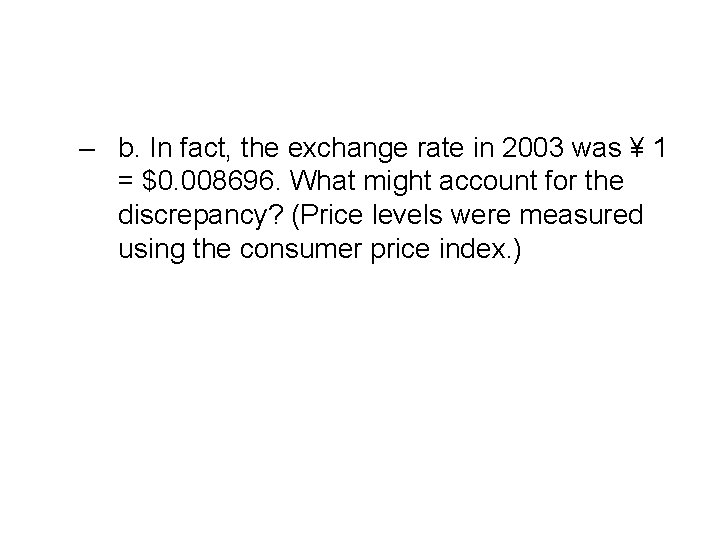– b. In fact, the exchange rate in 2003 was ¥ 1 = \$0. 008696. What might account for the discrepancy? (Price levels were measured using the consumer price index. )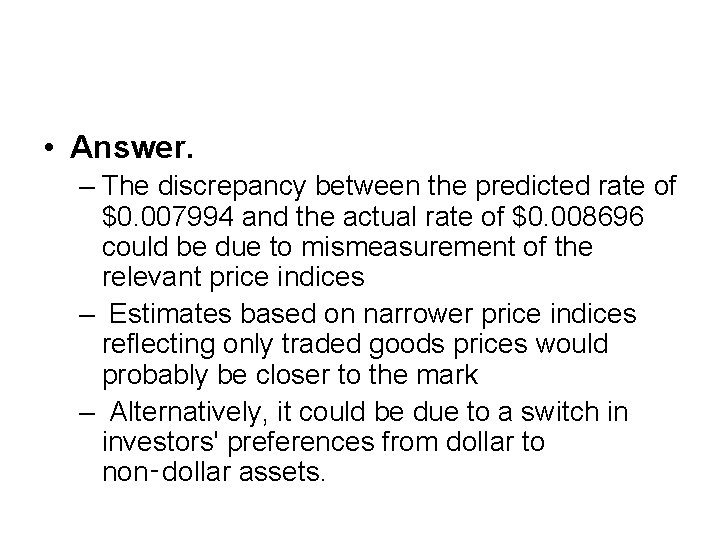• Answer. – The discrepancy between the predicted rate of \$0. 007994 and the actual rate of \$0. 008696 could be due to mismeasurement of the relevant price indices – Estimates based on narrower price indices reflecting only traded goods prices would probably be closer to the mark – Alternatively, it could be due to a switch in investors' preferences from dollar to non‑dollar assets.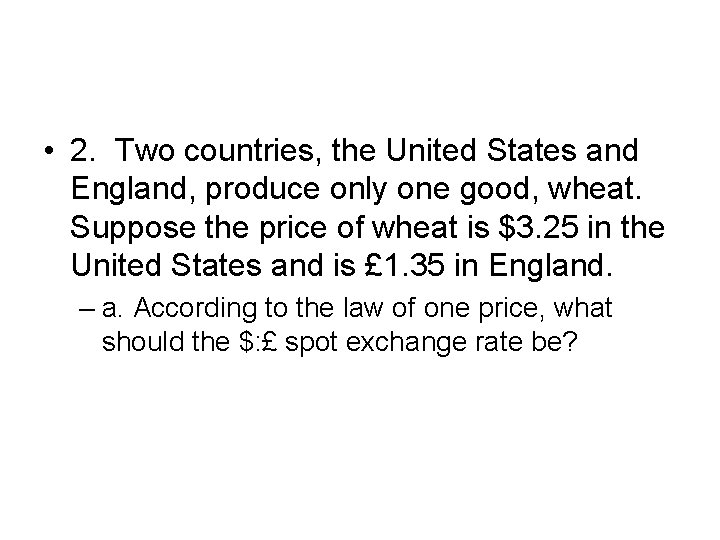• 2. Two countries, the United States and England, produce only one good, wheat. Suppose the price of wheat is \$3. 25 in the United States and is £ 1. 35 in England. – a. According to the law of one price, what should the \$: £ spot exchange rate be?• Answer. Since the price of wheat must be the same in both nations, the exchange rate, e, is 3. 25/1. 35 or e = \$2. 4074.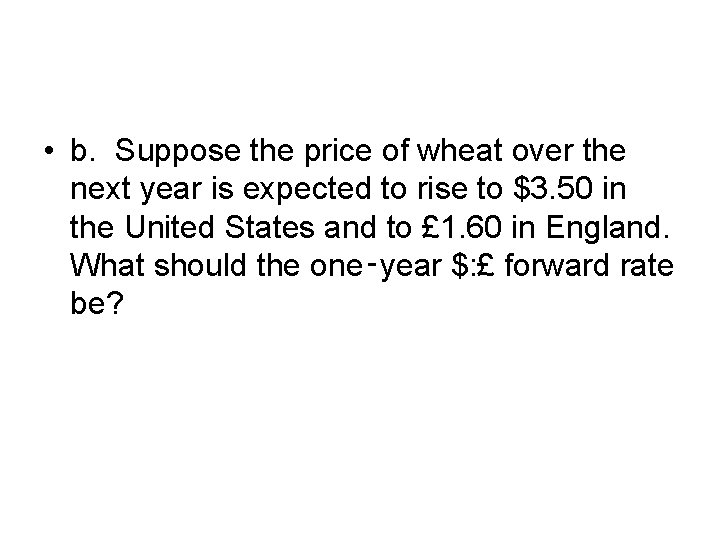• b. Suppose the price of wheat over the next year is expected to rise to \$3. 50 in the United States and to £ 1. 60 in England. What should the one‑year \$: £ forward rate be?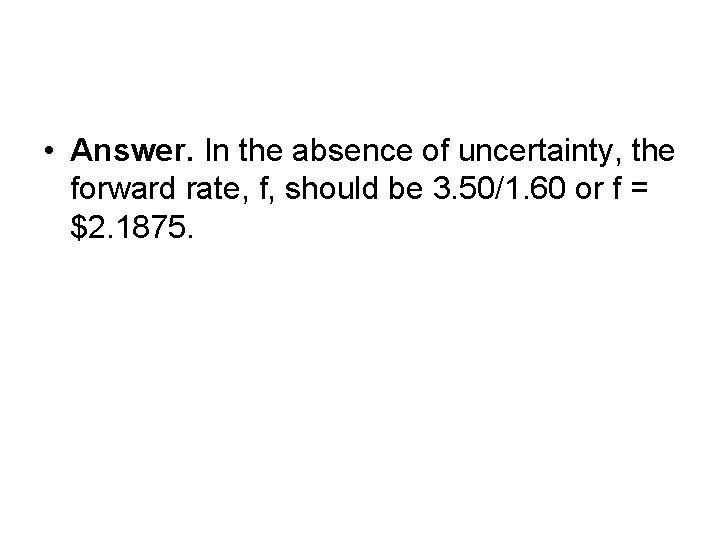• Answer. In the absence of uncertainty, the forward rate, f, should be 3. 50/1. 60 or f = \$2. 1875.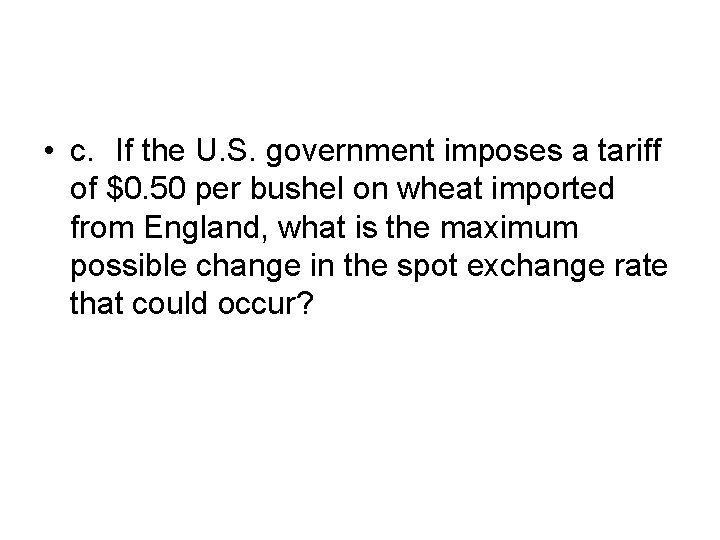• c. If the U. S. government imposes a tariff of \$0. 50 per bushel on wheat imported from England, what is the maximum possible change in the spot exchange rate that could occur?• Answer. If e is the exchange rate, then wheat selling in England at £ 1. 35 will sell in the United States for 1. 35 e + 0. 5, where 0. 5 is the U. S. tariff on English wheat. In order to eliminate the possibility of arbitrage, 1. 35 e + 0. 5 must be greater than or equal to \$3. 25, the price of wheat in the U. S. or e > \$2. 0370. Thus the maximum exchange rate change that could occur is (2. 4074 ‑ 2. 0370)/2. 4074 = 15. 38%. This solution assumes that the pound and dollar prices of wheat remain the same as before the tariff.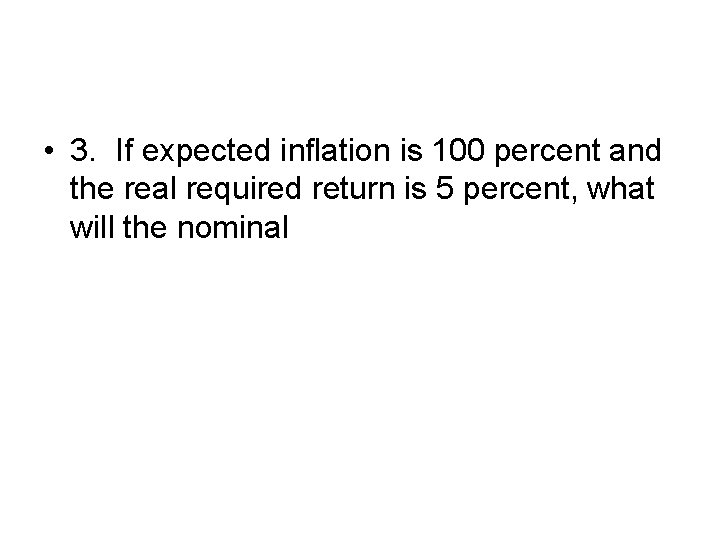• 3. If expected inflation is 100 percent and the real required return is 5 percent, what will the nominal• Answer. According to the Fisher effect, the relationship between the nominal interest rate, r, the real interest rate a, and the expected inflation rate, i, is 1 + r = (1 + a)(1 + i). Substituting in the numbers in the problem yields 1 + r = 1. 05 x 2 = 2. 1, or r = 110%.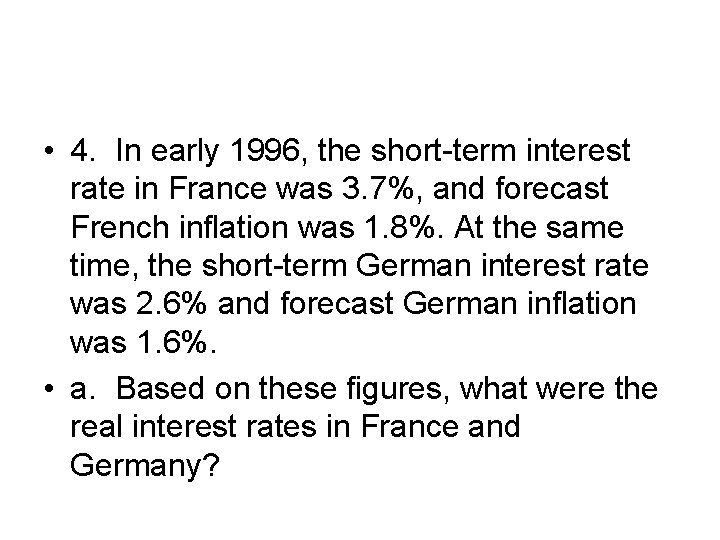• 4. In early 1996, the short-term interest rate in France was 3. 7%, and forecast French inflation was 1. 8%. At the same time, the short-term German interest rate was 2. 6% and forecast German inflation was 1. 6%. • a. Based on these figures, what were the real interest rates in France and Germany?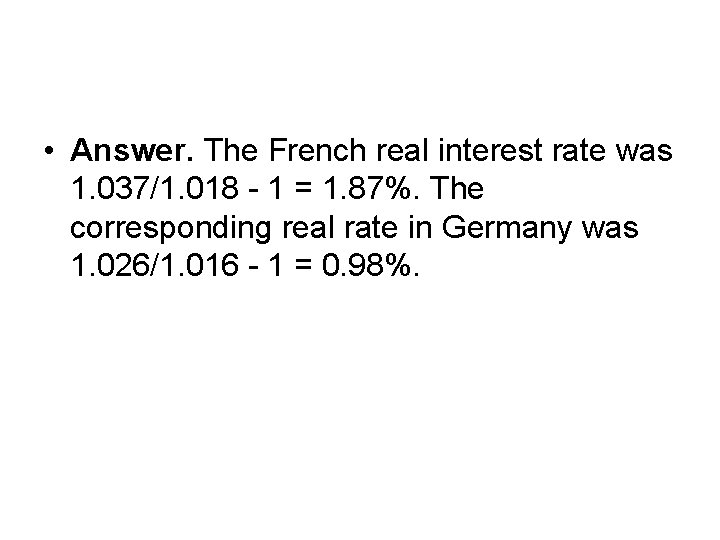• Answer. The French real interest rate was 1. 037/1. 018 - 1 = 1. 87%. The corresponding real rate in Germany was 1. 026/1. 016 - 1 = 0. 98%.• b. To what would you attribute any discrepancy in real rates between France and Germany?• Answer. • The most likely reason for the discrepancy is the inclusion of a higher inflation risk component in the French real interest rate than in the German real rate. • Other possibilities are the effects of currency risk or transactions costs precluding this seeming arbitrage opportunity.• 5. In July, the one‑year interest rate is 12% on British pounds and 9% on U. S. dollars. • a. If the current exchange rate is \$1. 63: £ 1, what is the expected future exchange rate in one year?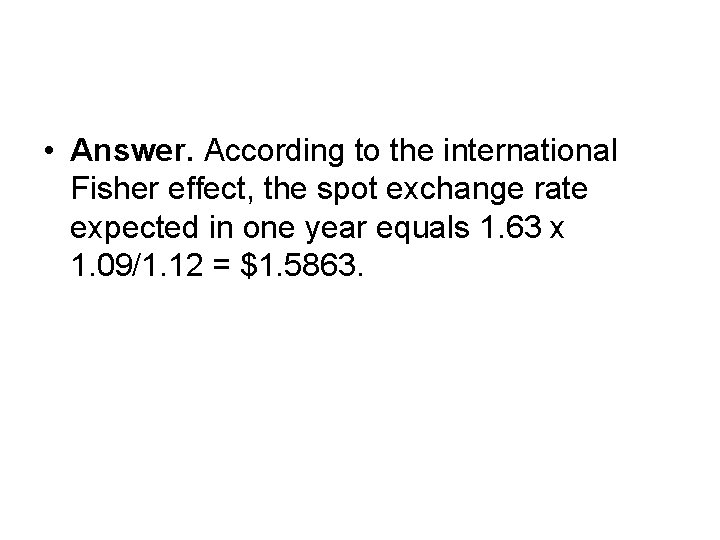• Answer. According to the international Fisher effect, the spot exchange rate expected in one year equals 1. 63 x 1. 09/1. 12 = \$1. 5863.• b. Suppose a change in expectations regarding future U. S. inflation causes the expected future spot rate to decline to \$1. 52: £ 1. What should happen to the U. S. interest rate?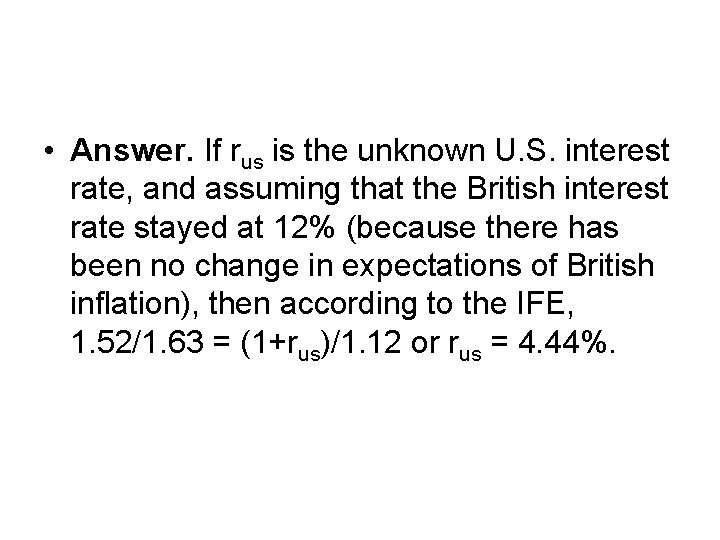• Answer. If rus is the unknown U. S. interest rate, and assuming that the British interest rate stayed at 12% (because there has been no change in expectations of British inflation), then according to the IFE, 1. 52/1. 63 = (1+rus)/1. 12 or rus = 4. 44%.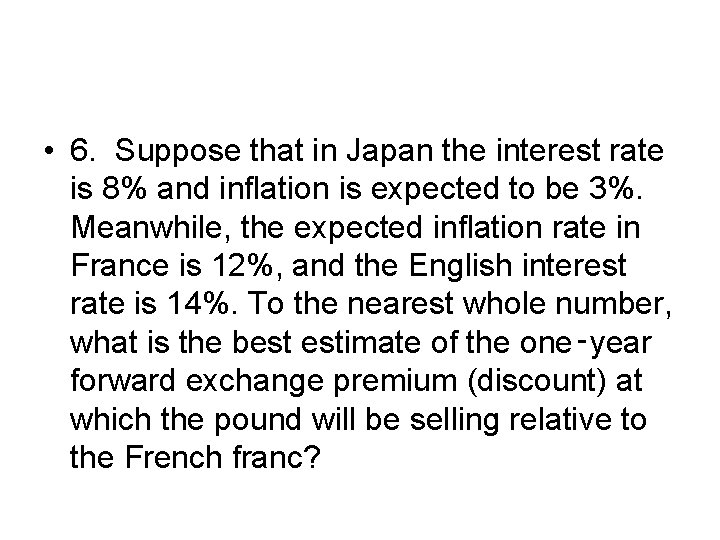• 6. Suppose that in Japan the interest rate is 8% and inflation is expected to be 3%. Meanwhile, the expected inflation rate in France is 12%, and the English interest rate is 14%. To the nearest whole number, what is the best estimate of the one‑year forward exchange premium (discount) at which the pound will be selling relative to the French franc?• Answer. Based on the numbers, Japan's real interest rate is about 5% (8% ‑ 3%). From that, we can calculate France's nominal interest rate as about 17% (12% + 5%), assuming that arbitrage will equate real interest rates across countries and currencies. Since England's nominal interest rate is 14%, for interest rate parity to hold, the pound should sell at around a 3% forward premium relative to the French franc.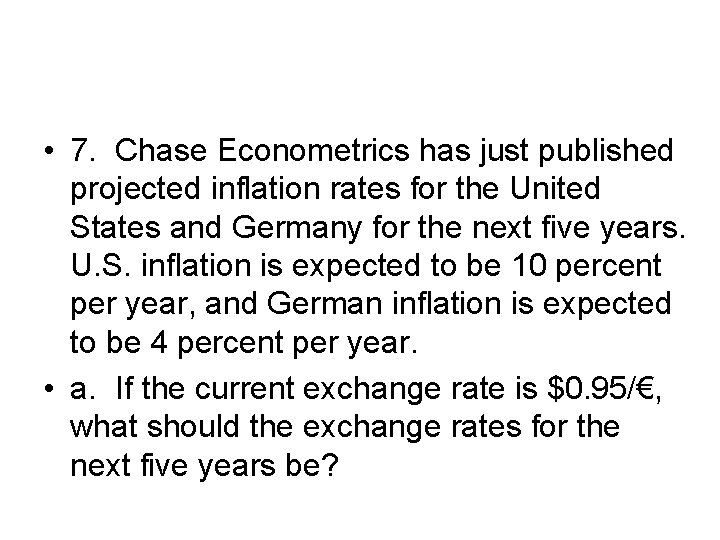• 7. Chase Econometrics has just published projected inflation rates for the United States and Germany for the next five years. U. S. inflation is expected to be 10 percent per year, and German inflation is expected to be 4 percent per year. • a. If the current exchange rate is \$0. 95/€, what should the exchange rates for the next five years be?• Answer. According to PPP, the exchange rate for the euro at the end of year t should equal 0. 95(1. 10/1. 04)t. Hence, projected exchange rates for the next 5 years are \$1. 0048, \$1. 0628, \$1. 1241, \$1. 1889, \$1. 2575.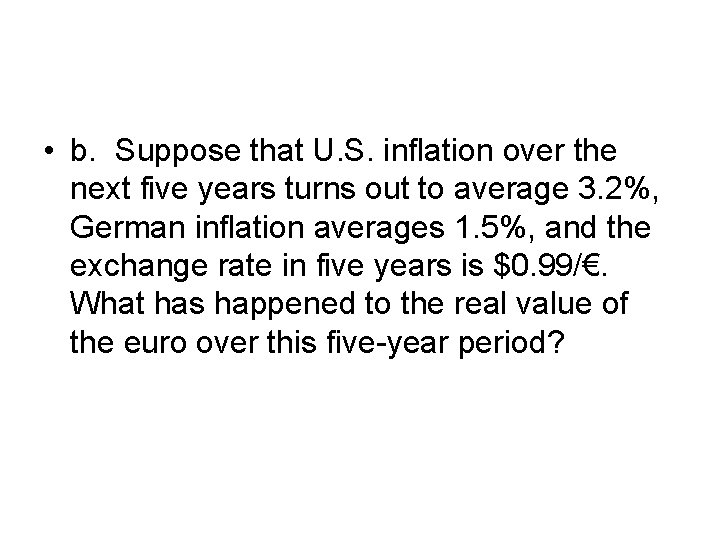• b. Suppose that U. S. inflation over the next five years turns out to average 3. 2%, German inflation averages 1. 5%, and the exchange rate in five years is \$0. 99/€. What has happened to the real value of the euro over this five-year period?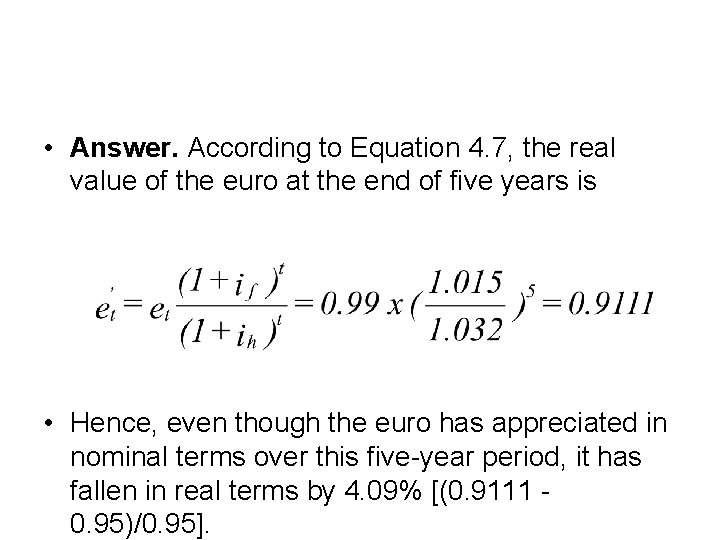• Answer. According to Equation 4. 7, the real value of the euro at the end of five years is • Hence, even though the euro has appreciated in nominal terms over this five-year period, it has fallen in real terms by 4. 09% [(0. 9111 0. 95)/0. 95].• 8. During 1995, the Mexican peso exchange rate rose from Mex\$5. 33/U. S. \$ to Mex\$7. 64/U. S. \$. At the same time, U. S. inflation was approximately 3% in contrast to Mexican inflation of about 48. 7%.• a. By how much did the nominal value of the peso change during 1995?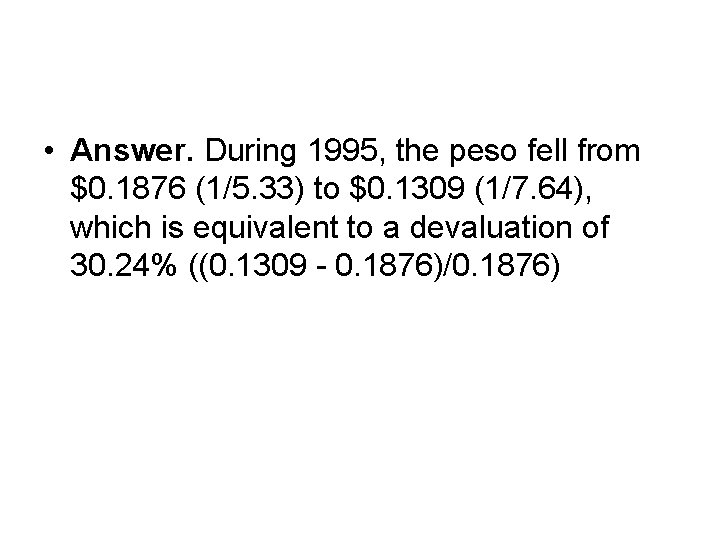• Answer. During 1995, the peso fell from \$0. 1876 (1/5. 33) to \$0. 1309 (1/7. 64), which is equivalent to a devaluation of 30. 24% ((0. 1309 - 0. 1876)/0. 1876)• b. By how much did the real value of the peso change over this period?• Based on this real exchange rate, the peso has appreciated during 1995 by 0. 72% ((0. 1890 0. 1876)/0. 1876). In other words, the real exchange rate stayed virtually constant, implying the purchasing power parity held during the year.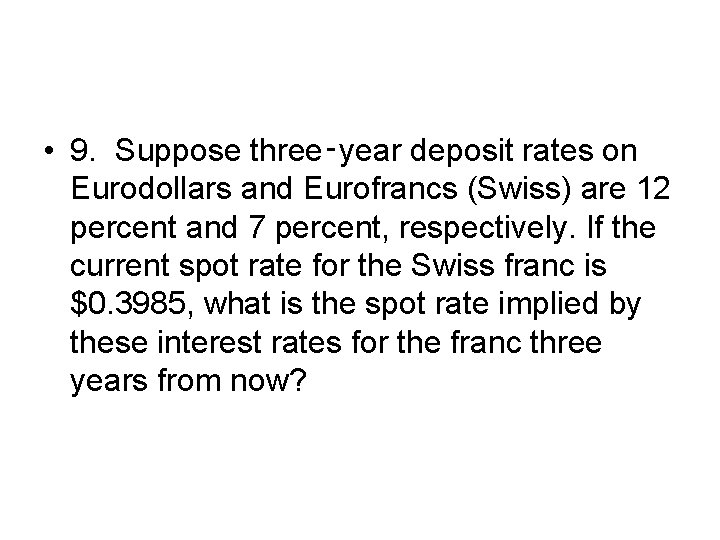• 9. Suppose three‑year deposit rates on Eurodollars and Eurofrancs (Swiss) are 12 percent and 7 percent, respectively. If the current spot rate for the Swiss franc is \$0. 3985, what is the spot rate implied by these interest rates for the franc three years from now?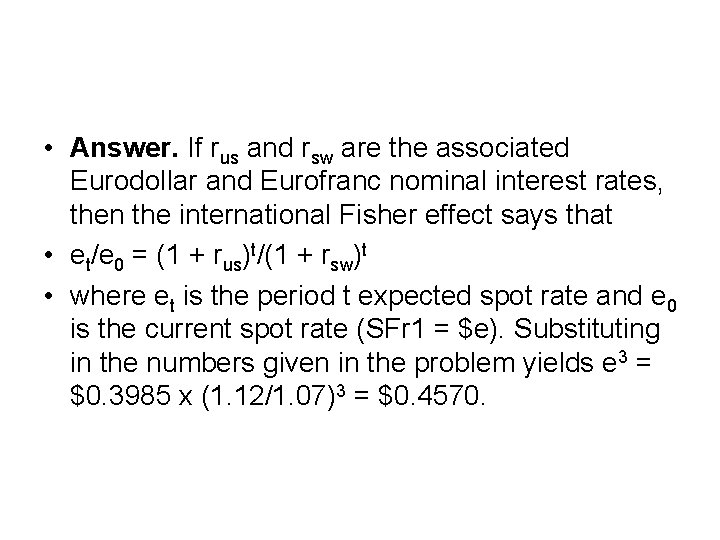• Answer. If rus and rsw are the associated Eurodollar and Eurofranc nominal interest rates, then the international Fisher effect says that • et/e 0 = (1 + rus)t/(1 + rsw)t • where et is the period t expected spot rate and e 0 is the current spot rate (SFr 1 = \$e). Substituting in the numbers given in the problem yields e 3 = \$0. 3985 x (1. 12/1. 07)3 = \$0. 4570.• 10. Assume the interest rate is 16 percent on pounds sterling and 7 percent on euros. At the same time, inflation is running at an annual rate of 3 percent in Germany and 9 percent in England. • a. If the euro is selling at a one-year forward premium of 10 percent against the pound, is there an arbitrage opportunity? Explain.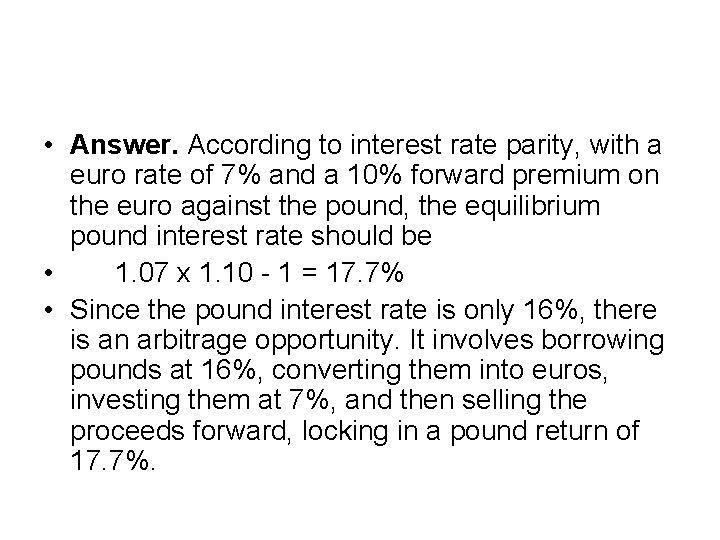• Answer. According to interest rate parity, with a euro rate of 7% and a 10% forward premium on the euro against the pound, the equilibrium pound interest rate should be • 1. 07 x 1. 10 - 1 = 17. 7% • Since the pound interest rate is only 16%, there is an arbitrage opportunity. It involves borrowing pounds at 16%, converting them into euros, investing them at 7%, and then selling the proceeds forward, locking in a pound return of 17. 7%.• b. What is the real interest rate in Germany? in England?• Answer. The real interest rate in Germany is 1. 07/1. 03 -1 = 3. 88%. The real interest rate in England is 1. 16/1. 09 -1 = 6. 42%.• c. Suppose that during the year the exchange rate changes from € 1. 8/£ 1 to € 1. 77/£ 1. What are the real costs to a German company of borrowing pounds? Contrast this cost to its real cost of borrowing euros.• Answer. At the end of one year, the German company must repay £ 1. 16 for every pound borrowed. However, since the pound has devalued against the euro by 1. 67% (1. 77/1. 80 - 1 = -1. 67%), the effective cost in euros is 1. 16 x (1 0. 0167) - 1 = 14. 07%. In real terms, given the 3% rate of German inflation, the cost of the pound loan is found as 1. 1407/1. 03 -1 = 10. 75%.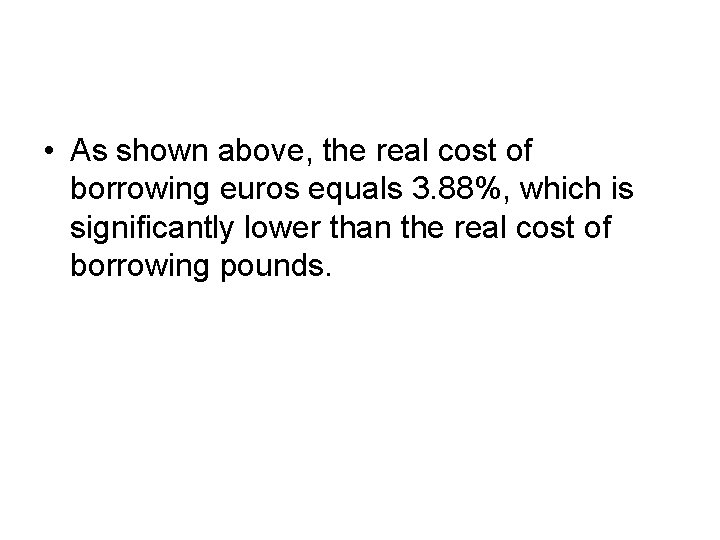• As shown above, the real cost of borrowing euros equals 3. 88%, which is significantly lower than the real cost of borrowing pounds.• d. What are the real costs to a British firm of borrowing euros? Contrast this cost to its real cost of borrowing pounds.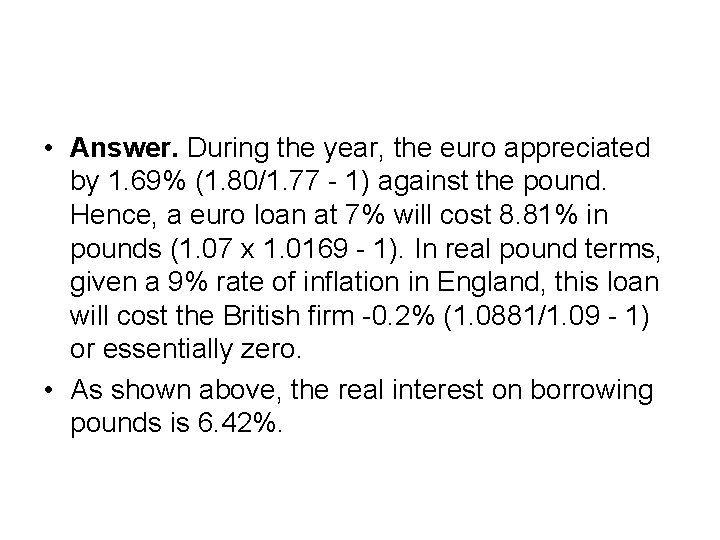• Answer. During the year, the euro appreciated by 1. 69% (1. 80/1. 77 - 1) against the pound. Hence, a euro loan at 7% will cost 8. 81% in pounds (1. 07 x 1. 0169 - 1). In real pound terms, given a 9% rate of inflation in England, this loan will cost the British firm -0. 2% (1. 0881/1. 09 - 1) or essentially zero. • As shown above, the real interest on borrowing pounds is 6. 42%.• 11. Suppose the Eurosterling rate is 15 percent, and the Eurodollar rate is 11. 5 percent. What is the forward premium on the dollar? Explain.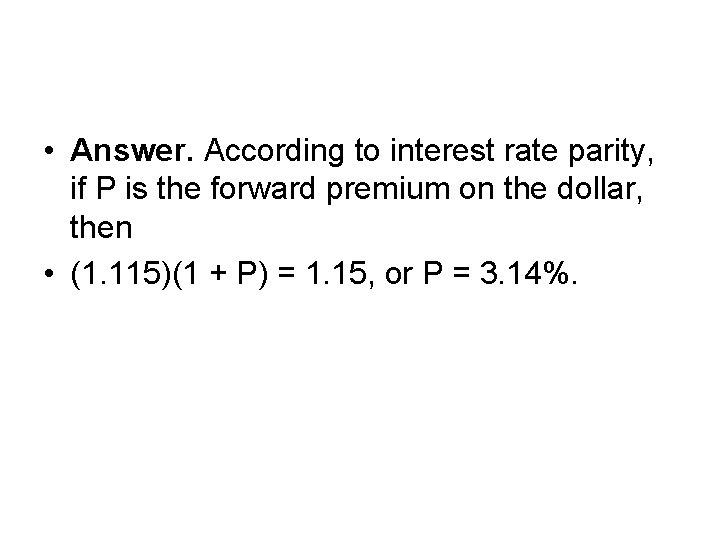• Answer. According to interest rate parity, if P is the forward premium on the dollar, then • (1. 115)(1 + P) = 1. 15, or P = 3. 14%.• 13. Suppose that three-month interest rates (annualized) in Japan and the United States are 7 percent and 9 percent, respectively. If the spot rate is ¥ 142: \$1 and the 90 -day forward rate is ¥ 139: \$1: • a. Where would you invest?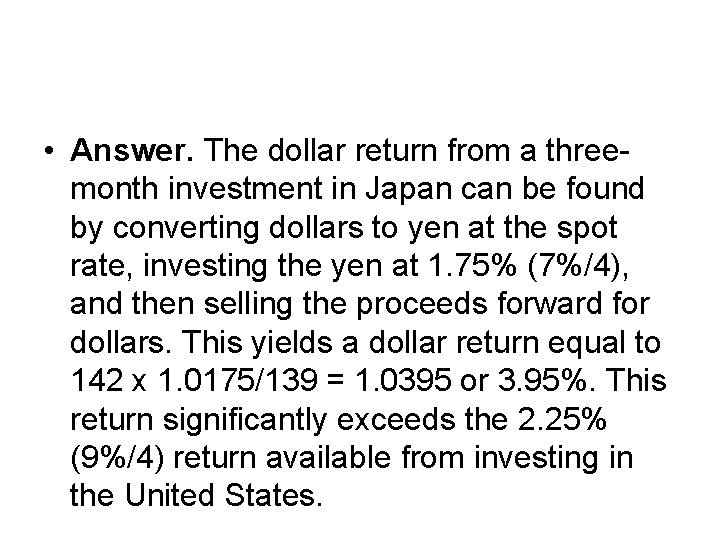• Answer. The dollar return from a threemonth investment in Japan can be found by converting dollars to yen at the spot rate, investing the yen at 1. 75% (7%/4), and then selling the proceeds forward for dollars. This yields a dollar return equal to 142 x 1. 0175/139 = 1. 0395 or 3. 95%. This return significantly exceeds the 2. 25% (9%/4) return available from investing in the United States.• b. Where would you borrow?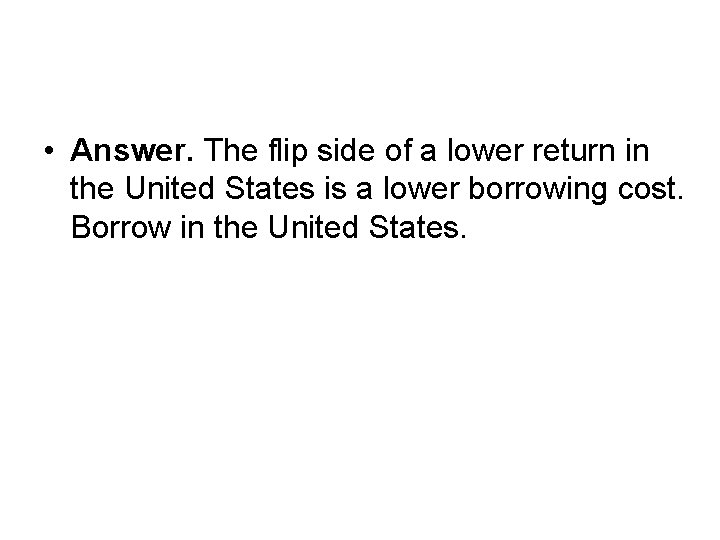• Answer. The flip side of a lower return in the United States is a lower borrowing cost. Borrow in the United States.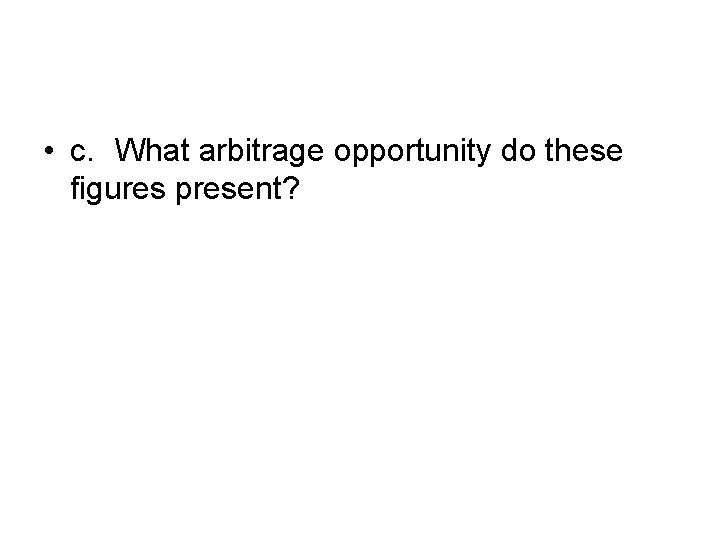• c. What arbitrage opportunity do these figures present?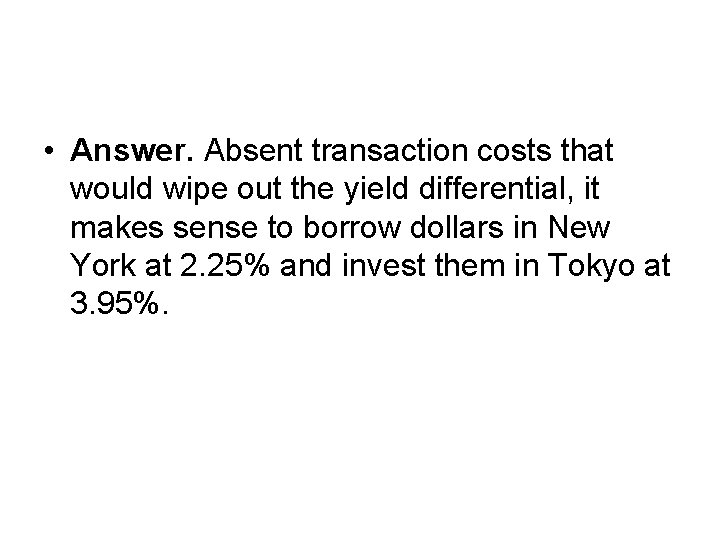• Answer. Absent transaction costs that would wipe out the yield differential, it makes sense to borrow dollars in New York at 2. 25% and invest them in Tokyo at 3. 95%.• d. Assuming no transaction costs, what would be your arbitrage profit per dollar or dollar-equivalent borrowed?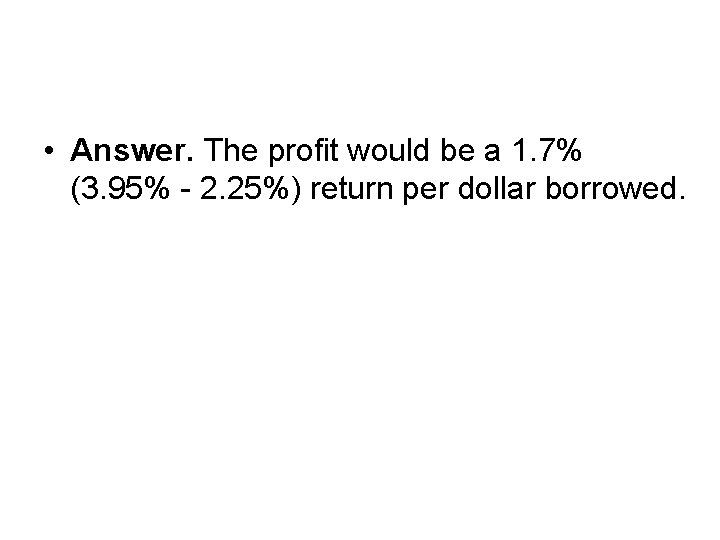• Answer. The profit would be a 1. 7% (3. 95% - 2. 25%) return per dollar borrowed.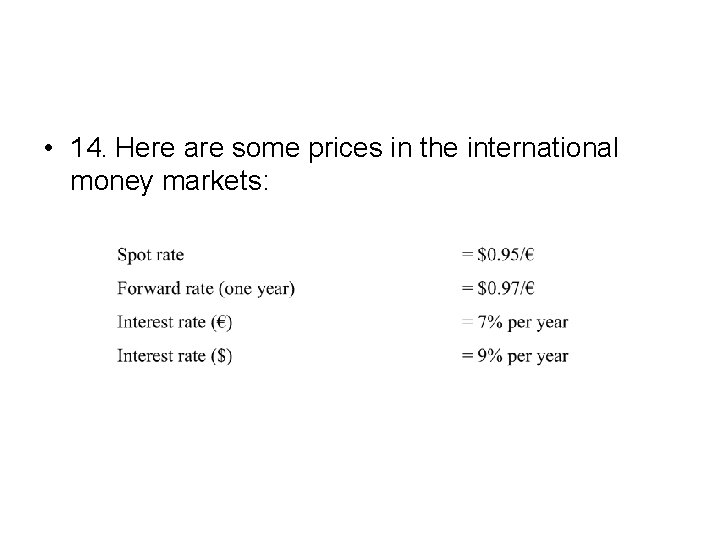• 14. Here are some prices in the international money markets: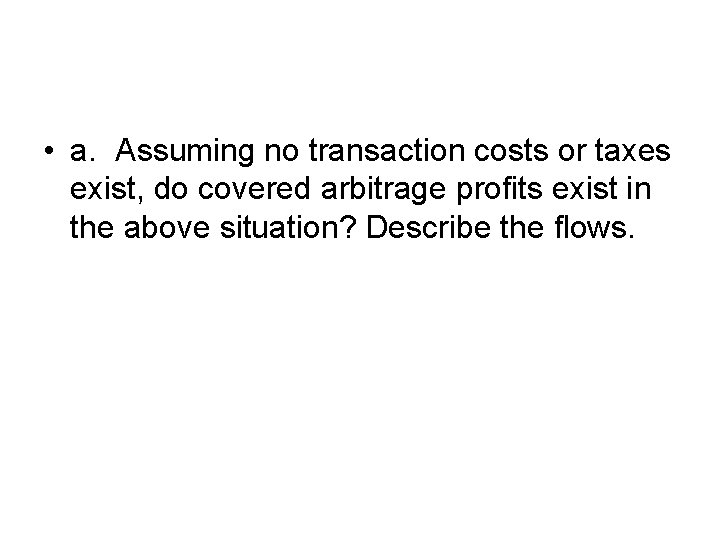• a. Assuming no transaction costs or taxes exist, do covered arbitrage profits exist in the above situation? Describe the flows.• Answer. The annual dollar return on dollars invested in Germany is (1. 07 x 0. 97)/0. 95 - 1 = 9. 25%. This return exceeds the 9% return on dollars invested in the United States by 0. 25% per annum. • Hence arbitrage profits can be earned by borrowing dollars or selling dollar assets, buying euros in the spot market, investing the euros at 7%, and simultaneously selling the euro interest and principal forward for one year for dollars.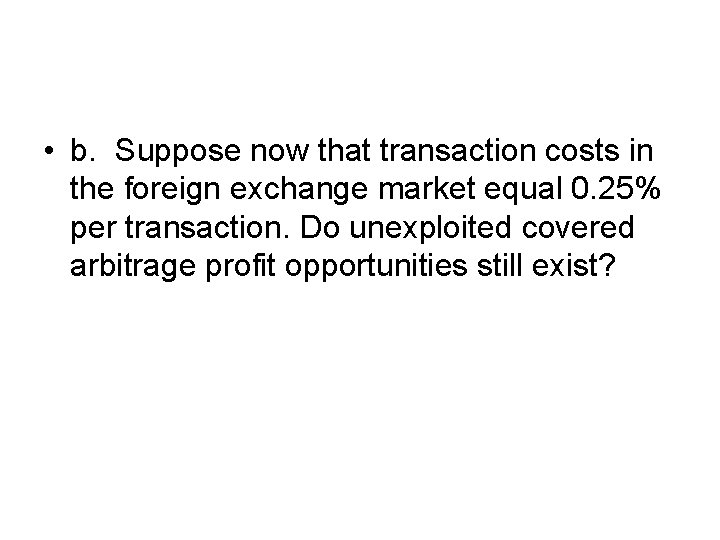• b. Suppose now that transaction costs in the foreign exchange market equal 0. 25% per transaction. Do unexploited covered arbitrage profit opportunities still exist?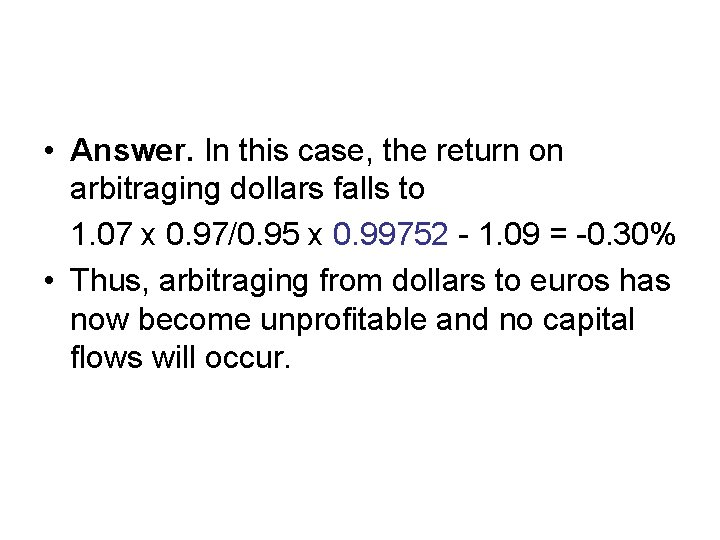• Answer. In this case, the return on arbitraging dollars falls to 1. 07 x 0. 97/0. 95 x 0. 99752 - 1. 09 = -0. 30% • Thus, arbitraging from dollars to euros has now become unprofitable and no capital flows will occur.• c. Suppose no transaction costs exist. Let the capital gains tax on currency profits equal 25%, and the ordinary income tax on interest income equal 50%. In this situation, do covered arbitrage profits exist? How large are they? Describe the transactions required to exploit these profits. (Hint: go back the formula of the interest rate parity)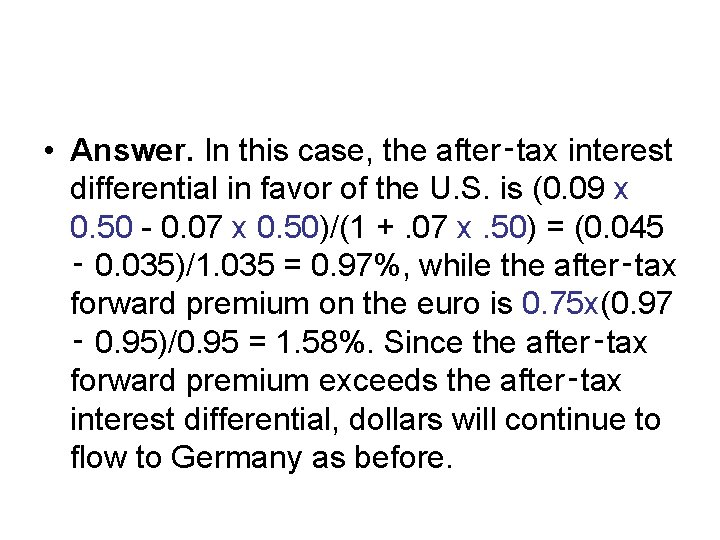• Answer. In this case, the after‑tax interest differential in favor of the U. S. is (0. 09 x 0. 50 - 0. 07 x 0. 50)/(1 +. 07 x. 50) = (0. 045 ‑ 0. 035)/1. 035 = 0. 97%, while the after‑tax forward premium on the euro is 0. 75 x(0. 97 ‑ 0. 95)/0. 95 = 1. 58%. Since the after‑tax forward premium exceeds the after‑tax interest differential, dollars will continue to flow to Germany as before.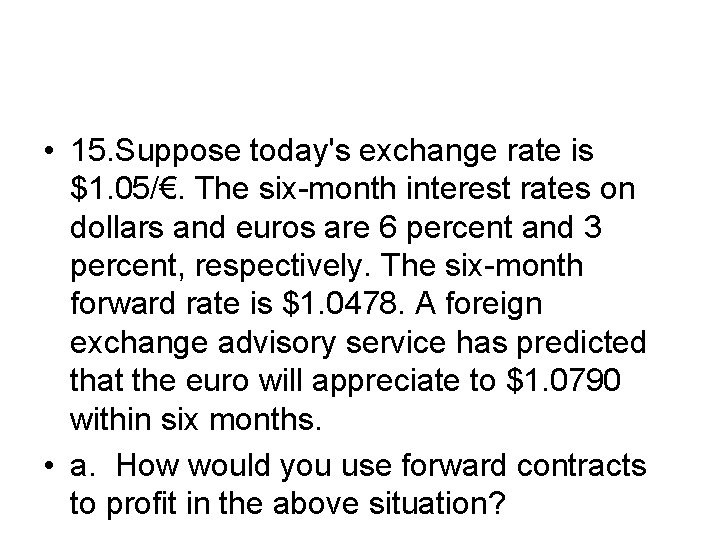• 15. Suppose today's exchange rate is \$1. 05/€. The six-month interest rates on dollars and euros are 6 percent and 3 percent, respectively. The six-month forward rate is \$1. 0478. A foreign exchange advisory service has predicted that the euro will appreciate to \$1. 0790 within six months. • a. How would you use forward contracts to profit in the above situation?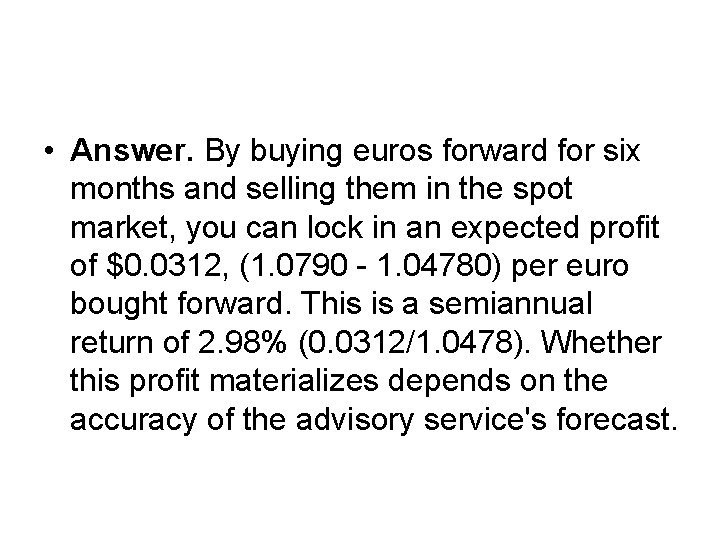• Answer. By buying euros forward for six months and selling them in the spot market, you can lock in an expected profit of \$0. 0312, (1. 0790 - 1. 04780) per euro bought forward. This is a semiannual return of 2. 98% (0. 0312/1. 0478). Whether this profit materializes depends on the accuracy of the advisory service's forecast.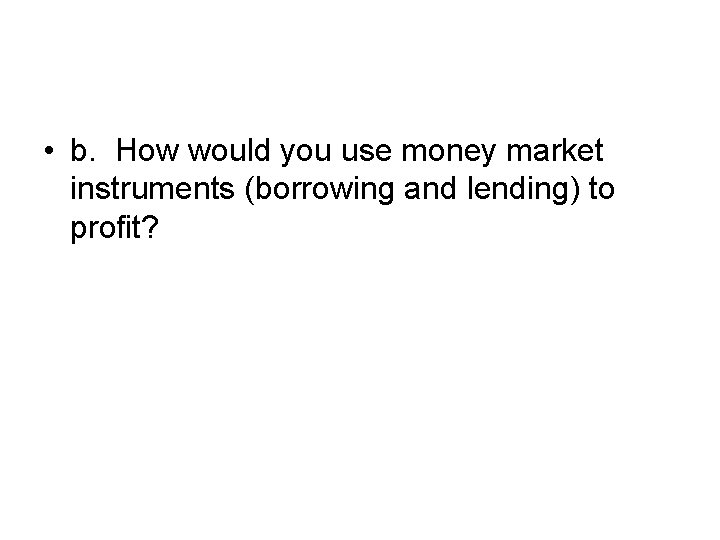• b. How would you use money market instruments (borrowing and lending) to profit?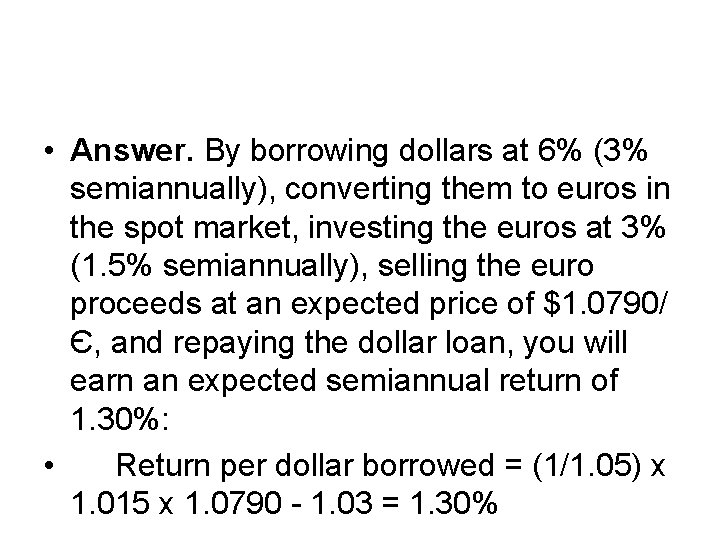• Answer. By borrowing dollars at 6% (3% semiannually), converting them to euros in the spot market, investing the euros at 3% (1. 5% semiannually), selling the euro proceeds at an expected price of \$1. 0790/ Є, and repaying the dollar loan, you will earn an expected semiannual return of 1. 30%: • Return per dollar borrowed = (1/1. 05) x 1. 015 x 1. 0790 - 1. 03 = 1. 30%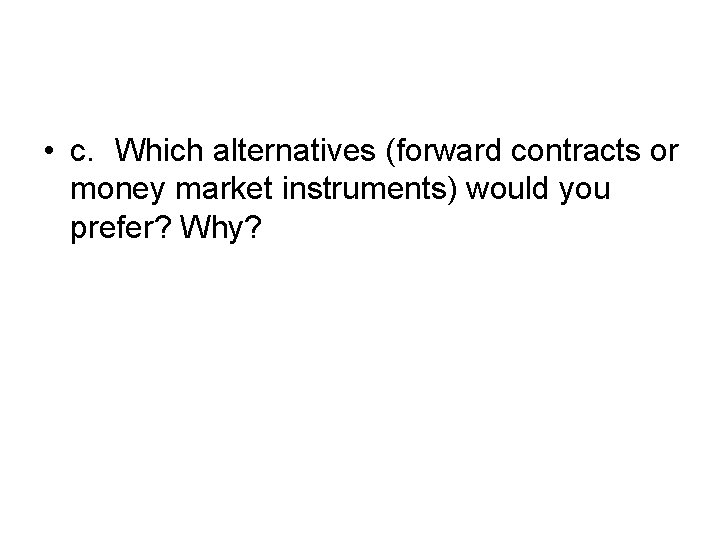• c. Which alternatives (forward contracts or money market instruments) would you prefer? Why?• Answer. The return per dollar in the forward market is substantially higher than the return using the money market speculation. Other things being equal, therefore, the forward market speculation would be preferred.An Additional Question (A General case with transaction cost) • American Airlines is trying to decide how to go about hedging SFr 70 million in ticket sales receivable in 180 days. Suppose it faces the following exchange and interest rates. Spot rate: \$0. 6433 -42/SFr Forward rate (180 days): \$0. 6578 -99/SFr 180 -day interest rate (annualized): 4. 01%-3. 97% U. S. dollar 180 -day interest rate (annualized): 8. 01%-7. 98%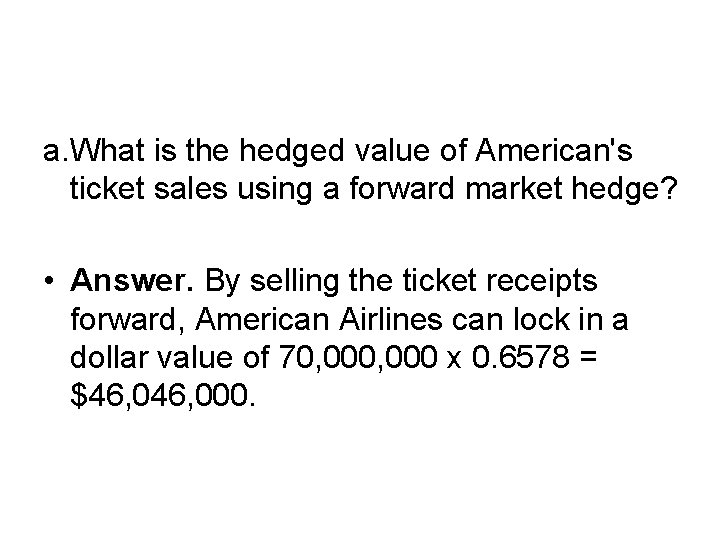a. What is the hedged value of American's ticket sales using a forward market hedge? • Answer. By selling the ticket receipts forward, American Airlines can lock in a dollar value of 70, 000 x 0. 6578 = \$46, 000.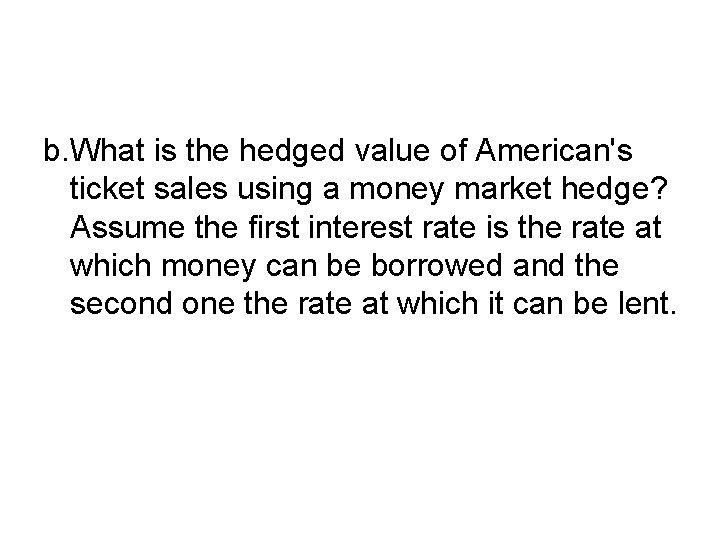b. What is the hedged value of American's ticket sales using a money market hedge? Assume the first interest rate is the rate at which money can be borrowed and the second one the rate at which it can be lent.• Answer. American also hedge it euro receivable by borrowing the present value of SFr 70 million at a 180 -day interest rate of 2. 005% (4. 01%/2), sell the proceeds in the spot market at a rate of \$0. 6433/SFr, and invest the dollar proceeds at a 180 -day interest rate of 3. 99% (7. 98%/2). Using this money market hedge, American lock in a value for its SFr 70 million receivable of \$45, 907, 296 (70, 000/1. 02005 x 0. 6433 x 1. 0399).c. Which hedge is less expensive? • Answer. The forward market hedge yields a higher dollar value for the ticket receivables, so it is preferable.d. Is there an arbitrage opportunity here? • Answer. Yes. By borrowing dollars at a semiannual rate of 4. 005% (8. 01%/2), converting them to euros at the ask rate of \$0. 6442, and simultaneously investing the euros at a semiannual rate of 1. 985% (3. 97%/2) and selling the loan proceeds forward at a bid rate of \$0. 6578, you can lock in an arbitrage spread of 0. 133% semiannually.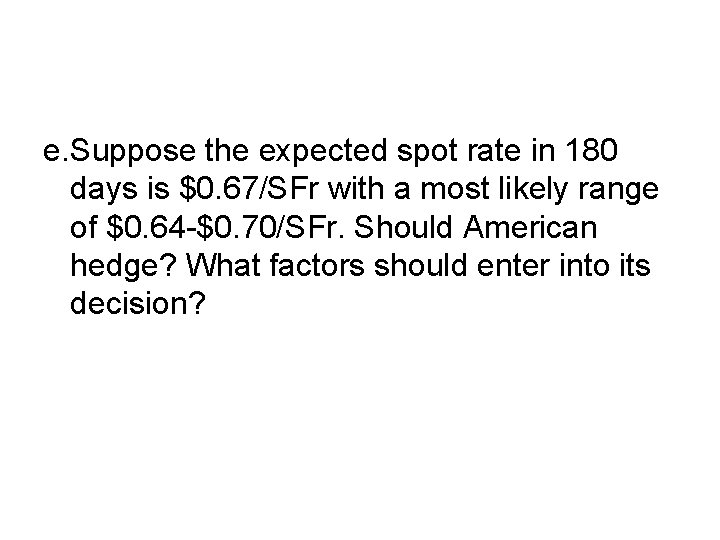e. Suppose the expected spot rate in 180 days is \$0. 67/SFr with a most likely range of \$0. 64 -\$0. 70/SFr. Should American hedge? What factors should enter into its decision?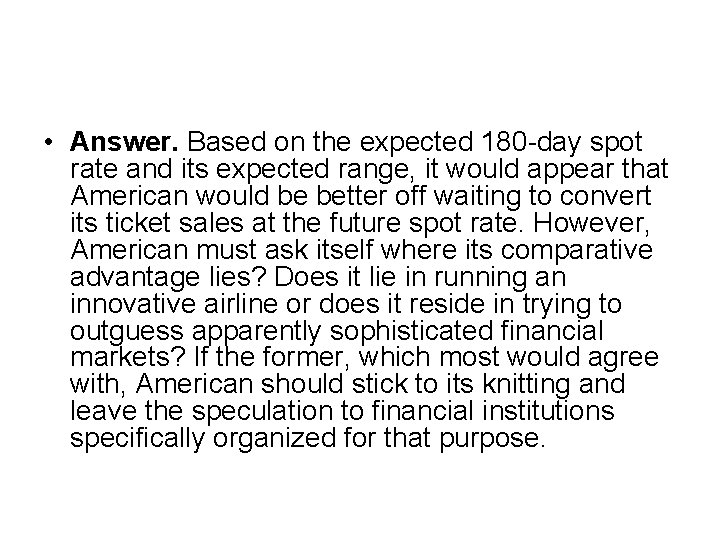• Answer. Based on the expected 180 -day spot rate and its expected range, it would appear that American would be better off waiting to convert its ticket sales at the future spot rate. However, American must ask itself where its comparative advantage lies? Does it lie in running an innovative airline or does it reside in trying to outguess apparently sophisticated financial markets? If the former, which most would agree with, American should stick to its knitting and leave the speculation to financial institutions specifically organized for that purpose.